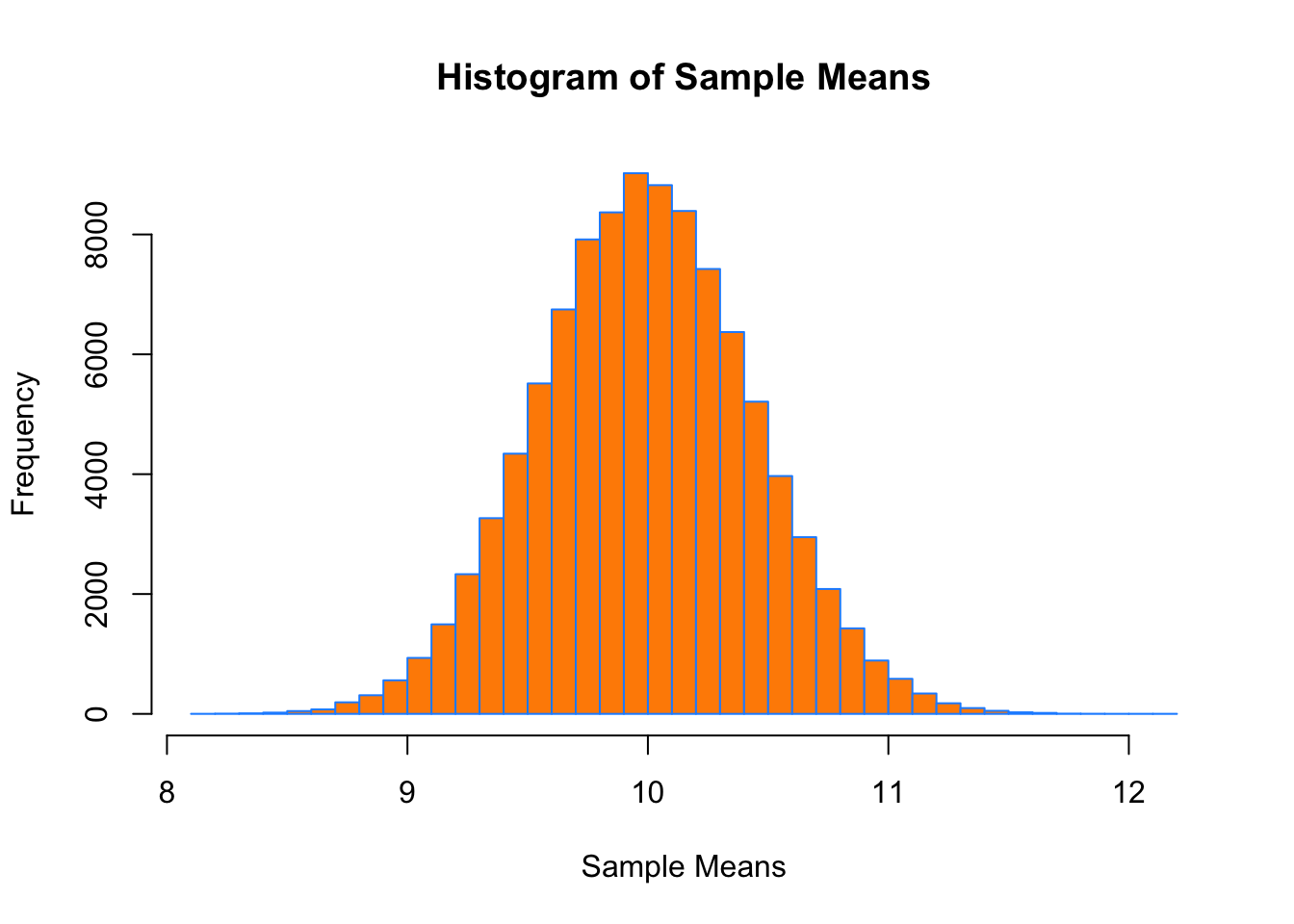Lab Goal: The goal of this lab is to introduce rmarkdown!

You can use the .Rmd file that created this document as a template for this lab.

# Markdown

[Exercise] Write an unordered list of your classes this semester. Make the short course name (STAT 430) bold. Have the short course name link to the course description in the course explorer. Make the actual course title (Basics of Statistical Learning) italic.

Solution:

• STAT 400 - Statistcis and Probability I
• STAT 430 - Basics of Statistical Learning

# Chunks

[Exercise] Add a chunk to this document that sums the integers from 1 to 100. It should display both the code and results after being knit. (Also, can you make this chunk only show output when knit? How about display the code, but don’t run the chunk when knit?)

Solution:

sum(1:100)
##  5050

Code Hidden:

##  5050

Not run:

sum(1:100)

# Style

The following code is “correct” but terrible. Don’t write code like this!

set.seed(1337);mu=10;sample_size=50;samples=100000;
x_bars=rep(0, samples)
for(i in 1:samples)
{
x_bars[i]=mean(rpois(sample_size,lambda = mu))}
x_bar_hist=hist(x_bars,breaks=50,main="Histogram of Sample Means",xlab="Sample Means",col="darkorange",border = "dodgerblue")
mean(x_bars>mu-2*sqrt(mu)/sqrt(sample_size)&x_bars<mu+2*sqrt(mu)/sqrt(sample_size))

[Exercise] Fix this code! You don’t need to change how the code accomplishes the task, but you should update the style. (Also, what is this code doing?)

Solution:

set.seed(1337)
mu = 10
sample_size = 50
samples = 100000
x_bars = rep(0, samples)

for (i in 1:samples) {
x_bars[i] = mean(rpois(sample_size, lambda = mu))
}

x_bar_hist = hist(x_bars,
breaks = 50,
main = "Histogram of Sample Means",
xlab = "Sample Means",
col = "darkorange",
border = "dodgerblue")mean(x_bars > mu - 2 * sqrt(mu) / sqrt(sample_size) &
x_bars < mu + 2 * sqrt(mu) / sqrt(sample_size))
##  0.95429

Some things that were “wrong” with that code:

• Line length too long.
• Use of ; is discouraged.
• Opening { should be on same lines as for()
• Lack of indentation.
• No spaces after each ,
• No spaces around binary operators.

We were essentially simulating the CLT and verifying the results.

# Randomization

rnorm(10)
set.seed(42)
rnorm(10)

[Exercise] Repeatedly run the (entire) two previous chunks. What’s the difference?

Solution:

The first changes each time. The second is the same each time. This is because we have set a seed.

# LaTeX

With RMarkdown, you can not only mix R and markdown, but you can also use LaTeX. It can be used inline: $$y = x + 2$$ or in “equation mode,”

$a^2 + b^2 = c^2$

[Exercise] Write the density function for a normal distribution using LaTeX in equation mode.

• Hint: You can obtain the LaTeX for any equation in R4SL by right clicking it, selecting “Show Math As” then clicking “TeX Commands.”

Solution:

$f(x | \mu, \sigma^2) = \frac{1}{\sigma\sqrt{2\pi}} \cdot \exp\left[\frac{-1}{2} \left(\frac{x - \mu}{\sigma}\right)^2 \right], \ \ \ -\infty < x < \infty, \ -\infty < \mu < \infty, \ \sigma > 0.$

# Chunk and Document Options

Some other things to try:

• Can you make the plot above larger?
• Can you change the theme of this document?

# After the Lab

After this lab, we’ll do some more live coding to briefly discuss:

• Functions
• Vectors vs Lists
• Data Frames
• Tibbles
• Working interactively with rmarkdown
• Importing data
• Why you should never, ever, set a working directory

:)